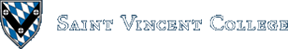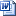Computing & Information Systems    DepartmentSearch CIS Site      Tutorials

### Spring 2020

This course has two main goals: to teach the student the basics of programming in a modern version of the Fortran language and to use that language to find numerical solutions to mathematical problems, especially problems of interest in mathematics, science, and engineering. In order to do this, a good deal of time is spent on studying elementary numerical analysis. Good software engineering techniques are emphasized in the programming portions of the course. Mathematica and hand calculators are used to help in solving several types of numerical analysis problems. However, the Fortran work that forms the bulk of the course is done on the CIS department's new Linux server, in part due to its speed. You can access this server most easily from any of the CIS lab PCs. You can also access this server from another computer on the campus network if you are not connected via wirelss and get appropriate software (such as SecureCRT, TeraTerm, or PuTTY) as described on the server usage page. Contact Br. David if you want to try connecting to this server from the dorms or from off-campus. You will need to supply your computer's IP address (external IP address if you are on a home network). Then we will grant access to that IP address. For some unknown reason, access via wireless in Dupre and possible elsewhere does not work.

### Further Information

 The Course Syllabus Grading Guidelines Hidden Figures the book and movie show early computers -- people -- who calculated orbits and learned to program -- in Fortran. Documents, homilies, and speeches of Pope Francis Poverty, Hunger, and Social Justice in AmericaIn honor of Martin Luther King, Jr. Wolfram Mathematica Documentation Center Wolfram Learning Center Wolfram MathWorldContains a wealth of resources, including some on algebra and number theory. Wolfram Functions Wolfram Library Wolfram Demonstrations Education web site by Texas Instruments Includes manuals and tutorials for many TI calculators and links to math web sites. Information on UNIX Editors For information on available commands on our Linux server, enter the following at the comand prompt `topic` `topic 12` See the Server Usage page for information about our server and the software you can use to access it. The easiest way to compile a Fortran 90 program on our Linux system is as follows, where we assume that the program is named `series.f90`: `build90 series` The `build90` script will link in the library of numerical subroutines if needed. It will put the executable in a file with the name `series` (without the .f90). You then run the program by entering `./series` at the command prompt. Information on Well-known Series Power Series Of interest especially to engineering students: ACM Code of Ethics Fortran programs: Anyone can copy these examples when viewing them in a browser. Students in this class can also copy them at the command line as in the following two examples: `cp /home/cs270/trap1.f90 .` (The dot is needed and means the current directory. Thus we copy the file to the current directory and keep the same filename.) `cp /home/cs270/convert.f90 myconvert.f90` (This will copy to a new filename in the current directory.) aboveavg.f90 Illustrates the use of a 1-dimensional array. arith0.f90 Does a division, multiplies the quotient by the denominator, and sometimes does not get the numerator exactly. arith1.f90 Prints the largest integer, the largest plus 1, etc. arith2.f90 Tries division by zero, etc. arith3.f90 Experiments with adding large and small numbers, as well as adding in different orders. arith4.f90 Reports on characteristics of integers and reals. arith5.f90 Reports on characteristics of integers and reals of various kinds. average1.f90 Averages a list of numbers with 0 used to end data entry. average2.f90 Uses a WHILE loop to solve the same problem. bsectst1.f90 Uses bisection algorithm to find a zero of a function. bsectst2.f90 bsectst3.f90 convert.f90 Simple program to convert from Fahrenheit to Kelvin. deriv1.f90 Program to compute a derivative at a point. epsilon1.f90 Estimates the machine epsilon. epsilon2.f90 A minor variation on the previous program. epsilon3.f90 Shows how to use a subroutine. factorial.f90 Prints the factorial of each nonnegative integer entered. integ3.f90 Uses the numerical subroutine library to find a good approximation for a definite integral. leqtest.f90 Uses Gaussian elimination with partial pivoting to solve a system of linear equations. leq.in Input data file for the previous program. linear1.f90 Uses a library subroutine to solve a system of linear equations. linear2.f90 Solves a system of 3 linear equations in 3 variables via simple-minded elimination and back substitution. looptest.f90 Finds the limits for integer and real loop control variables. machine1.f90 Looks up and prints the values of various machine constants. machine2.f90 Looks up and prints the values of some other machine constants. matrices1.f90 Adds 2-dimensional matrices using a couple of methods. matrices2.f90 Finds a matrix product as well as the product of a matrix and a column vector. max.f90 Finds the maximum number in a list of real inputs and finds the location of that maximum. maxmin.f90 Finds where max and min are in a list of integers. multiply1.f90Simply multiplies two numbers. multiply2.f90 Prints some products and quotients. newton3.f90 Uses Newton's method for finding a zero of a function. newraph3.f90 Uses Newton-Raphson method for finding a zero of a function. ode1.f90 Solves an ordinary differential equation via Euler's method. ode2.f90 Solves another ordinary differential equation via Euler's method. ode3.f90 Uses Taylor's series to solve an ordinary differential equation. ode4.f90 Uses Taylor's series to solve another ordinary differential equation. quadeq1a.f90 Solves a quadratic equation via the quadratic formula. quadeq2.f90 Solves a quadratic equation, but adds the scaling of the coefficients and the avoidance of possible catastrophic cancellation that blind application of the quadratic formula can give. readfile.dat Data file to accompany the following program. readfile.f90 Shows how to read numbers from a formatted file. series1.f90 Finds a partial sum of the series 1+ 1/2 + 1/4 + 1/8 +... series2.f90 Finds sin(x) for a particular value x by using the power series for sin(x). series2dbl.f90 A version of the previous example, but using double precision reals. series3.f90 Uses an array so that terms can be added from smallest to largest. series8.f90 Program to investigate a Fourier series that converges to 1 on (-1, 1), to 0 at 1, to -1 on (1, 3), etc. series15.f90 Uses a power series approximation to find an approximate value for a definite integral. SeriesToFile.f90 Program to get partial sums of the geometric series using data read from a file. Output goes to a file. sort.f90 An insertion sort for data in a linear array. sqroot.f90 Prints a table of square roots. More importantly, it shows how to use a 1-dimensional array. subroutines.f90 Illustrates how to write and use subroutines. Really short subroutines are used in this and the next two examples in order to keep the examples simple. subroutines2.f90 Illustrates how to write and use subroutines. This one has a subroutine with an INOUT parameter. subroutines3.f90 Illustrates how to write and use subroutines. This one replaces one of the subroutines in the previous two examples with a function. trap1.f90 Uses the trapezoidal rule to find an approximate value for a definite integral. trap2.f90 Double precision version of trap1.f90. trebuchet.f90 Given a starting speed and a user input for the angle of elevation, this program finds how long a rock hurled by a trebuchet would be in the air and how far it would travel horizontally. Air resistance is ignored.

### Examples and Class Materials

 Medieval WarfareIn class we may use this PBS material on the trebuchet to produce a program that calculates how far a trebuchet might be able to hurl a large rock. Ariane 5 Rocket Explosion Video of the Ariane 5 Explosion NumericalComputations.pdf Solving quadratic equations (especially in Fortran) Solving quadratic equations with Mathematica. Holistic Numerical Methods Institute Taylor's Theorem Fourier Series: Basic Results Illustration of Eigenvalues and Eigenvectors Series in Mathematics a summary of series with links to additional information Real Analysis, an interactive online textbook Rounding according to the IEEE floating point standard.

### Homework and Announcements

 See Schoology

#### Instructor: Br. David Carlson

Maintained by: Br. David Carlson
Last updated: January 21, 2020
Disclaimer